• 机器学习：SVM（核函数、高斯核函数RBF） 一、核函数（Kernel Function） 　1）格式 K(x, y)：表示样本 x 和 y，添加多项式特征得到新的样本 x'、y'，K(x, y) 就是返回新的样本经过计算得到的值； 在 SVM 类型的...
转载自博客园：https://www.cnblogs.com/volcao/p/9465214.html

SVM（核函数、高斯核函数RBF）

一、核函数（Kernel Function）

1）格式

K(x, y)：表示样本 x 和 y，添加多项式特征得到新的样本 x'、y'，K(x, y) 就是返回新的样本经过计算得到的值；
在 SVM 类型的算法 SVC() 中，K(x, y) 返回点乘：x' . y' 得到的值；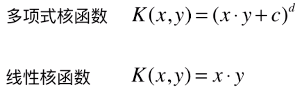2）多项式核函数

业务问题：怎么分类非线性可分的样本的分类？

内部实现：

对传入的样本数据点添加多项式项；
新的样本数据点进行点乘，返回点乘结果；
多项式特征的基本原理：依靠升维使得原本线性不可分的数据线性可分；
升维的意义：使得原本线性不可分的数据线性可分；

例：

一维特征的样本，两种类型，分布如图，线性不可分：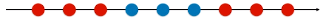为样本添加一个特征：x2 ，使得样本在二维平面内分布，此时样本在 x 轴升的分布位置不变；如图，可以线性可分：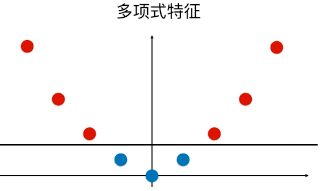3）优点 / 特点

不需要每次都具体计算出原始样本点映射的新的无穷维度的样本点，直接使用映射后的新的样本点的点乘计算公式即可；
减少计算量
减少存储空间
一般将原始样本变形，通常是将低维的样本数据变为高维数据，存储高维数据花费较多的存储空间；使用核函数，不用考虑原来样本改变后的样子，也不用存储变化后的结果，只需要直接使用变化的结果进行运算并返回运算结果即可；
核函数的方法和思路不是 SVM 算法特有，只要可以减少计算量和存储空间，都可以设计核函数方便运算；
对于比较传统的常用的机器学习算法，核函数这种技巧更多的在 SVM 算法中使用；
4）SVM 中的核函数

svm 类中的 SVC() 算法中包含两种核函数：
SVC(kernel = 'ploy')：表示算法使用多项式核函数；
SVC(kernel = 'rbf')：表示算法使用高斯核函数；
SVM 算法的本质就是求解目标函数的最优化问题；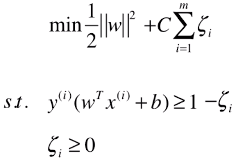求解最优化问题时，将数学模型变形：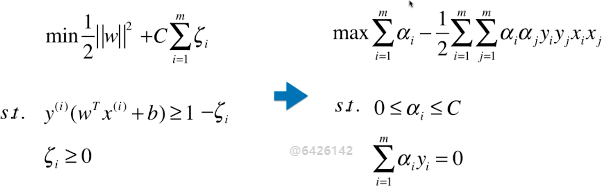5）多项式核函数

格式：

from sklearn.svm import SVC

svc = SVC(kernel = 'ploy')

思路：设计一个函数（ K(xi, xj) ），传入原始样本（x(i) 、 x(j)），返回添加了多项式特征后的新样本的计算结果（x'(i) . x'(j)）；
内部过程：先对 xi 、xj 添加多项式，得到：x'(i) 、 x'(j) ，再进行运算：x'(i) . x'(j) ；
x(i) 添加多项式特征后：x'(i) ；
x(j) 添加多项式特征后：x'(j) ；
x(i) . x(j) 转化为：x'(i) . x'(j) ；
其实不使用核函数也能达到同样的目的，这里核函数相当于一个技巧，更方便运算；
二、高斯核函数（RBF）

业务问题：怎么分类非线性可分的样本的分类？
1）思想

业务的目的是样本分类，采用的方法：按一定规律统一改变样本的特征数据得到新的样本，新的样本按新的特征数据能更好的分类，由于新的样本的特征数据与原始样本的特征数据呈一定规律的对应关系，因此根据新的样本的分布及分类情况，得出原始样本的分类情况。
应该是试验反馈，将样本的特征数据按一定规律统一改变后，同类样本更好的凝聚在了一起；
高斯核和多项式核干的事情截然不同的，如果对于样本数量少，特征多的数据集，高斯核相当于对样本降维；
高斯核的任务：找到更有利分类任务的新的空间。
方法：类似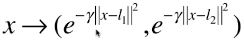的映射。

高斯核本质是在衡量样本和样本之间的“相似度”，在一个刻画“相似度”的空间中，让同类样本更好的聚在一起，进而线性可分。
疑问：
“衡量”的手段，经过这种映射之后，为什么同类样本能更好的分布在一起？

2）定义方式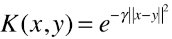；
x、y：样本或向量；
γ：超参数；高斯核函数唯一的超参数；
|| x - y ||：表示向量的范数，可以理解为向量的模；
表示两个向量之间的关系，结果为一个具体值；
高斯核函数的定义公式就是进行点乘的计算公式；
3）功能

先将原始的数据点（x， y）映射为新的样本（x'，y'）；
再将新的特征向量点乘（x' . y'），返回其点乘结果；
计算点积的原因：此处只针对 SVM 中的应用，在其它算法中的应用不一定需要计算点积；4）特点

高斯核运行开销耗时较大，训练时间较长；
一般使用场景：数据集 (m, n)，m < n；
一般应用领域：自然语言处理；
自然语言处理：通常会构建非常高维的特征空间，但有时候样本数量并不多；

5）高斯函数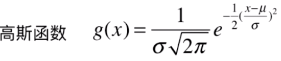正态分布就是一个高斯函数；
高斯函数和高斯核函数，形式类似；

6）其它

高斯核函数，也称为 RBF 核（Radial Basis Function Kernel），也称为径向基函数；
高斯核函数的本质：将每一个样本点映射到一个无穷维的特征空间；
无穷维：将 m*n 的数据集，映射为 m*m 的数据集，m 表示样本个数，n 表示原始样本特征种类，样本个数是无穷的，因此，得到的新的数据集的样本也是无穷维的；
高斯核升维的本质，使得线性不可分的数据线性可分；

三、RBF 转化特征数据原理

1）转化原理x：需要改变维度的样本；
np.array([l1, l2, ..., lm]) == X == np.array([x1, x2, ... , xm])：Landmark，地标，一般直接选取数据集 X 的所有样本作为地标；（共 m 个）
对于 (m, n) 的数据集：转化为 (m, m)  的数据集；将 n 维的样本转化为 m 维的样本；
对于原始数据集中的每一个样本 x，也可以有几个地标点，就将 x 转化为几维；

2）主要为两部分

先将原始的数据点映射为一种新的特征向量，再将新的特征向量点乘，返回其点乘结果；

维度转化：样本 x1 转化 x1' ：(e-γ||x1 - x1||**2, e-γ||x1 - x2||**2, e-γ||x1 - x3||**2, ..., e-γ||x1 - xm||**2)，同理样本 x2 的转化 x2' ；（地标点就是数据集 X 的样本点）
点乘计算：x1' . x2' == K(x1, x2) == e-γ||x1 - x2||**2，最终结果为一个具体值；

3）实例模拟维度转化过程

一维升到二维
原始样本分布：第一步：选取地标点：L1、L2 ；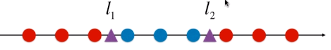第二步：升维计算四、程序模拟

目的：将线性不可分的数据变为线性可分；
方法：一维数据升到二维；
1）模拟数据集

x 数据集：每一个样本只有一个特征，且分布规律线性不可分；
np.arange(m, n, l)：将区间 [m, n) 按间距为 l 等分，等分后的数据点包含 m 值，不包含 n；
*len(x[y==0])： 是一个 list，list * C 表示将列表复制 C 份；
如：*5 == [0, 0, 0, 0, 0]import numpy as np
import matplotlib.pyplot as plt

x = np.arange(-4, 5, 1)
y = np.array((x >= -2) & (x <= 2), dtype='int')

plt.scatter(x[y==0], *len(x[y==0]))
plt.scatter(x[y==1], *len(x[y==1]))
plt.show()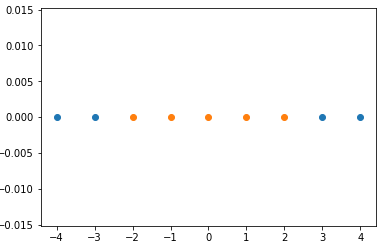2）经过高斯核，得到新的数据集

np.exp(m)：表示 e 的 m 次幂；
np.empty(元组)：（元组）=(m, n)，生成一个 m 行 n 列的空的矩阵；
enumerate(iterator)：返回可迭代对象的 index 和 value；
for i, data in enumerate(x)：i 存放向量 x 的 index，data 存放向量 x 的 index 对应的元素值；def gaussian(x, l):
# 此处直接将超参数 γ 设定为 1.0；
# 此处 x 表示一维的样本，也就是一个具体的值，l 相应的也是一个具体的数，因为 l 和 x 一样，从特征空间中选定；
gamma = 1.0
# 此处因为 x 和 l 都只是一个数，不需要再计算模，可以直接平方；
return np.exp(-gamma * (x-l)**2)

# 设定地标 l1、l2 为 -1和1
l1, l2 = -1, 1
x_new = np.empty((len(x), 2))

for i, data in enumerate(x):
x_new[i, 0] = gaussian(data, l1)
x_new[i, 1] = gaussian(data, l2)

plt.scatter(x_new[y==0, 0], x_new[y==0, 1])
plt.scatter(x_new[y==1, 0], x_new[y==1, 1])
plt.show()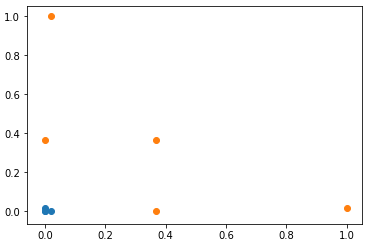展开全文机器学习
• 关于SVM中线性核函数和高斯核函数选择1.基本数据准备2.各情况对比1. SVM(kernel='linear')：直接采用数据集[966,1850]2. SVM(kernel='rbf')：直接采用数据集[966,1850]3. LR：直接采用数据集[966,1850]4. 降维之后...
Table of Contents
关于SVM中线性核函数和高斯核函数的选择1.基本数据准备2.各情况对比1. SVM(kernel='linear')：直接采用数据集[966,1850]2. SVM(kernel='rbf')：直接采用数据集[966,1850]3. LR：直接采用数据集[966,1850]4. 降维之后3.小结
关于SVM中线性核函数和高斯核函数的选择
SVM中常用核函数一般是线性核函数和高斯核函数。以sklearn中的SVC，提供的’linear’和’rbf’做说明。面向[n,m]原始数据集，一般的选取准则：

相对于n，m很大。比如m≥n, m=10000, n=10~1000,即(m/n)>10。
考虑’linear’
m很小，n一般大小。比如m=1-1000, n=10~10000,即(m/n)在[0.0001,100].
考虑’rbf’
m很小，n很大。比如n=1-1000，m=50000+，即(m/n)在[~,0.02].
增加m的量，考虑’linear’

补充：logistic约等同于’linear’的选择
#!/usr/bin/python
# encoding: utf-8

"""
@author : jack_lu
@File : SVM
@time : 2018/12/12 12:12
"""

# 练习所用数据集
from sklearn.datasets import fetch_lfw_people,olivetti_faces

# 特征提取方法
from sklearn.decomposition import PCA
from sklearn.discriminant_analysis import LinearDiscriminantAnalysis
# 特征转换方法
from sklearn.preprocessing import StandardScaler

# sklearn模型方法
from sklearn.model_selection import train_test_split

# metric方法
from sklearn.metrics import accuracy_score

# 机器学习模型
from sklearn.linear_model import LogisticRegression
from sklearn.pipeline import Pipeline
from sklearn.svm import SVC

1.基本数据准备
print('#'*50 + '  1.基本数据准备  ' + '#'*50)
lfw_people2 = fetch_lfw_people(min_faces_per_person=70, resize=0.4)  # 需要通过翻墙下载，C:\Users\Administrator\scikit_learn_data\lfw_home\joblib\sklearn\datasets\lfw\_fetch_lfw_people

##################################################  1.基本数据准备  ##################################################

n_samples, h, w = lfw_people2.images.shape
X = lfw_people2.data
y = lfw_people2.target
n_features = X.shape

target_names = lfw_people2.target_names
n_class = target_names.shape

print('#'*20,'数据集基本情况','#'*20)
print('**样本数量**：%d' %(X.shape))
print('**特征维度**：%d' %(X.shape))
print('**目标类别数**：%d' %(n_class))
print('#'*20,'数据集基本情况','#'*20)


#################### 数据集基本情况 ####################
**样本数量**：1288
**特征维度**：1850
**目标类别数**：7
#################### 数据集基本情况 ####################

X_train,X_test,y_train,y_test = train_test_split(X, y, test_size=0.25,random_state=1)

print('#'*20,'训练数据集基本情况','#'*20)
print('**训练样本数量**：%d' %(X_train.shape))
print('**训练特征维度**：%d' %(X_train.shape))
print('**目标类别数**：%d' %(n_class))
print('#'*20,'训练数据集基本情况','#'*20)


#################### 训练数据集基本情况 ####################
**训练样本数量**：966
**训练特征维度**：1850
**目标类别数**：7
#################### 训练数据集基本情况 ####################

2.各情况对比
print('#'*50 + '  2.建模与比较  ' + '#'*50)


##################################################  2.建模与比较  ##################################################

1. SVM(kernel=‘linear’)：直接采用数据集[966,1850]
svm_origin = SVC(kernel='linear', C=1000, decision_function_shape='ovo')  # 根据官方说明，对于多分类任务宜采用'ovo'即onevsone策略
svm_origin.fit(X_train, y_train)
y_pred = svm_origin.predict(X_test)
print('**情况1-linear的准确率**: %s' %(accuracy_score(y_pred=y_pred, y_true=y_test)))

**情况1-linear的准确率**: 0.832298136646

2. SVM(kernel=‘rbf’)：直接采用数据集[966,1850]
svm_rbf = SVC(kernel='rbf', C=1000, decision_function_shape='ovo')  # 根据官方说明，对于多分类任务宜采用'ovo'即onevsone策略
svm_rbf.fit(X_train, y_train)
y_pred = svm_rbf.predict(X_test)
print('**情况2-rbf的准确率**: %s' %(accuracy_score(y_pred=y_pred, y_true=y_test)))

**情况2-rbf的准确率**: 0.44099378882

3. LR：直接采用数据集[966,1850]
lr_origin = LogisticRegression()  # 对于多分类任务，multi_class可选择'ovr'或者'auto'自动选择，这里按照默认'auto'
lr_origin.fit(X_train, y_train)
y_pred = lr_origin.predict(X_test)
print('**情况3-LR的准确率**: %s' %(accuracy_score(y_pred=y_pred, y_true=y_test)))

**情况3-LR的准确率**: 0.826086956522

4. 降维之后
print('#'*20,'维度由1850减少到150之后','#'*20)


#################### 维度由1850减少到150之后 ####################

def namestr(obj, namespace):
return [name for name in namespace if namespace[name] is obj]
print(namestr(lr_origin,globals()),'\n',
namestr(lr_origin,globals()))


['lr_origin', 'model']
lr_origin

def small_feature_model(model,X_train=X_train,y_train=y_train,X_test=X_test, y_test=y_test):
pca = PCA(n_components=150,random_state=0,whiten=True)
pipeline = Pipeline([('scale',StandardScaler()),('pca',pca)])
processing = pipeline.fit(X_train)
X_train = processing.transform(X_train)
X_test = processing.transform(X_test)
model.fit(X_train, y_train)
y_pred = model.predict(X_test)
#     print(namestr(model,globals()))
print('**small-%s的准确率**: %.3f' %(namestr(model, globals()),accuracy_score(y_pred=y_pred, y_true=y_test)))

for model in [svm_origin, svm_rbf, lr_origin]:
small_feature_model(model)

**small-svm_origin的准确率**: 0.789
**small-svm_rbf的准确率**: 0.811
**small-lr_origin的准确率**: 0.835

print('#'*50 + '  完成  ' + '#'*50)


##################################################  完成  ##################################################

3.小结
从结果看到：

将维度减少到150之后，选择kernel='rbf’的效果>‘linear’;
在没有调参情况下，LR的效果还不错,初期建模值得先尝试。

当然，上面是指定了特定的参数，更主要的目的是对比SVM两种核方法在n和m的比例当中的效果。在具体问题当中，在计算力有限条件下，建议还是通过网格搜索的方法对比选出最优的kernel。


展开全文• 一、核函数（Kernel Function） 　1）格式 K(x, y)：表示样本 x 和 y，添加多项式特征得到新的样本 x'、y'，K(x, y) 就是返回新的样本经过计算得到的值； 在 SVM 类型的算法 SVC() 中，K(x, y) 返回点乘：x' .y...

一、核函数（Kernel Function）
1）格式
K(x, y)：表示样本 x 和 y，添加多项式特征得到新的样本 x'、y'，K(x, y) 就是返回新的样本经过计算得到的值；
在 SVM 类型的算法 SVC() 中，K(x, y) 返回点乘：x' . y' 得到的值；2）多项式核函数
业务问题：怎么分类非线性可分的样本的分类？

内部实现：

对传入的样本数据点添加多项式项；
新的样本数据点进行点乘，返回点乘结果；
多项式特征的基本原理：依靠升维使得原本线性不可分的数据线性可分；
升维的意义：使得原本线性不可分的数据线性可分；

例：

一维特征的样本，两种类型，分布如图，线性不可分：为样本添加一个特征：x2 ，使得样本在二维平面内分布，此时样本在 x 轴升的分布位置不变；如图，可以线性可分：3）优点 / 特点
不需要每次都具体计算出原始样本点映射的新的无穷维度的样本点，直接使用映射后的新的样本点的点乘计算公式即可；
减少计算量
减少存储空间
一般将原始样本变形，通常是将低维的样本数据变为高维数据，存储高维数据花费较多的存储空间；使用核函数，不用考虑原来样本改变后的样子，也不用存储变化后的结果，只需要直接使用变化的结果进行运算并返回运算结果即可；

核函数的方法和思路不是 SVM 算法特有，只要可以减少计算量和存储空间，都可以设计核函数方便运算；
对于比较传统的常用的机器学习算法，核函数这种技巧更多的在 SVM 算法中使用；

4）SVM 中的核函数
svm 类中的 SVC() 算法中包含两种核函数：
SVC(kernel = 'ploy')：表示算法使用多项式核函数；
SVC(kernel = 'rbf')：表示算法使用高斯核函数；
SVM 算法的本质就是求解目标函数的最优化问题；求解最优化问题时，将数学模型变形：5）多项式核函数

格式：

from sklearn.svm import SVC

svc = SVC(kernel = 'ploy')

思路：设计一个函数（ K(xi, xj) ），传入原始样本（x(i) 、 x(j)），返回添加了多项式特征后的新样本的计算结果（x'(i) . x'(j)）；
内部过程：先对 xi 、xj 添加多项式，得到：x'(i) 、 x'(j) ，再进行运算：x'(i) . x'(j) ；
x(i) 添加多项式特征后：x'(i) ；
x(j) 添加多项式特征后：x'(j) ；
x(i) . x(j)转化为：x'(i) . x'(j) ；
其实不使用核函数也能达到同样的目的，这里核函数相当于一个技巧，更方便运算；

二、高斯核函数（RBF）
业务问题：怎么分类非线性可分的样本的分类？
1）思想
业务的目的是样本分类，采用的方法：按一定规律统一改变样本的特征数据得到新的样本，新的样本按新的特征数据能更好的分类，由于新的样本的特征数据与原始样本的特征数据呈一定规律的对应关系，因此根据新的样本的分布及分类情况，得出原始样本的分类情况。
应该是试验反馈，将样本的特征数据按一定规律统一改变后，同类样本更好的凝聚在了一起；
高斯核和多项式核干的事情截然不同的，如果对于样本数量少，特征多的数据集，高斯核相当于对样本降维；
高斯核的任务：找到更有利分类任务的新的空间。
方法：类似的映射。

高斯核本质是在衡量样本和样本之间的“相似度”，在一个刻画“相似度”的空间中，让同类样本更好的聚在一起，进而线性可分。
疑问：
“衡量”的手段，经过这种映射之后，为什么同类样本能更好的分布在一起？

2）定义方式；
x、y：样本或向量；
γ：超参数；高斯核函数唯一的超参数；
|| x - y ||：表示向量的范数，可以理解为向量的模；
表示两个向量之间的关系，结果为一个具体值；
高斯核函数的定义公式就是进行点乘的计算公式；

3）功能
先将原始的数据点（x， y）映射为新的样本（x'，y'）；
再将新的特征向量点乘（x' . y'），返回其点乘结果；
计算点积的原因：此处只针对 SVM 中的应用，在其它算法中的应用不一定需要计算点积；4）特点
高斯核运行开销耗时较大，训练时间较长；
一般使用场景：数据集 (m, n)，m < n；
一般应用领域：自然语言处理；
自然语言处理：通常会构建非常高维的特征空间，但有时候样本数量并不多；

5）高斯函数正态分布就是一个高斯函数；
高斯函数和高斯核函数，形式类似；

6）其它
高斯核函数，也称为 RBF 核（Radial Basis Function Kernel），也称为径向基函数；
高斯核函数的本质：将每一个样本点映射到一个无穷维的特征空间；
无穷维：将 m*n 的数据集，映射为 m*m 的数据集，m 表示样本个数，n 表示原始样本特征种类，样本个数是无穷的，因此，得到的新的数据集的样本也是无穷维的；
高斯核升维的本质，使得线性不可分的数据线性可分；

三、RBF 转化特征数据原理
1）转化原理x：需要改变维度的样本；
np.array([l1, l2, ..., lm]) == X == np.array([x1, x2, ... , xm])：Landmark，地标，一般直接选取数据集 X 的所有样本作为地标；（共 m 个）
对于 (m, n) 的数据集：转化为 (m, m)  的数据集；将 n 维的样本转化为 m 维的样本；
对于原始数据集中的每一个样本 x，也可以有几个地标点，就将 x 转化为几维；

2）主要为两部分
先将原始的数据点映射为一种新的特征向量，再将新的特征向量点乘，返回其点乘结果；

维度转化：样本 x1 转化 x1' ：(e-γ||x1 - x1||**2, e-γ||x1 - x2||**2, e-γ||x1 - x3||**2, ..., e-γ||x1 - xm||**2)，同理样本 x2 的转化 x2' ；（地标点就是数据集 X 的样本点）
点乘计算：x1' . x2' == K(x1, x2) == e-γ||x1 - x2||**2，最终结果为一个具体值；

3）实例模拟维度转化过程
一维升到二维
原始样本分布：第一步：选取地标点：L1、L2 ；第二步：升维计算四、程序模拟
目的：将线性不可分的数据变为线性可分；
方法：一维数据升到二维；
1）模拟数据集
x 数据集：每一个样本只有一个特征，且分布规律线性不可分；
np.arange(m, n, l)：将区间 [m, n) 按间距为 l 等分，等分后的数据点包含 m 值，不包含 n；
*len(x[y==0])： 是一个 list，list * C 表示将列表复制 C 份；
如：*5 == [0, 0, 0, 0, 0]

import numpy as np
import matplotlib.pyplot as plt

x = np.arange(-4, 5, 1)
y = np.array((x >= -2) & (x <= 2), dtype='int')

plt.scatter(x[y==0], *len(x[y==0]))
plt.scatter(x[y==1], *len(x[y==1]))
plt.show()2）经过高斯核，得到新的数据集
np.exp(m)：表示 e 的 m 次幂；
np.empty(元组)：（元组）=(m, n)，生成一个 m 行 n 列的空的矩阵；
enumerate(iterator)：返回可迭代对象的 index 和 value；
for i, data in enumerate(x)：i 存放向量 x 的 index，data 存放向量 x 的 index 对应的元素值；

def gaussian(x, l):
# 此处直接将超参数 γ 设定为 1.0；
# 此处 x 表示一维的样本，也就是一个具体的值，l 相应的也是一个具体的数，因为 l 和 x 一样，从特征空间中选定；
gamma = 1.0
# 此处因为 x 和 l 都只是一个数，不需要再计算模，可以直接平方；
return np.exp(-gamma * (x-l)**2)

# 设定地标 l1、l2 为 -1和1
l1, l2 = -1, 1
x_new = np.empty((len(x), 2))

for i, data in enumerate(x):
x_new[i, 0] = gaussian(data, l1)
x_new[i, 1] = gaussian(data, l2)

plt.scatter(x_new[y==0, 0], x_new[y==0, 1])
plt.scatter(x_new[y==1, 0], x_new[y==1, 1])
plt.show()转载于:https://www.cnblogs.com/volcao/p/9465214.html
展开全文• SVM核函数选择对于其性能的表现有至关重要的作用，尤其是针对那些线性不可分的数据，因此核函数选择SVM算法中就显得至关重要。对于核技巧我们知道，其目的是希望通过将输入空间内线性不可分的数据映射到一个高...
SVM核函数的选择对于其性能的表现有至关重要的作用，尤其是针对那些线性不可分的数据，因此核函数的选择在SVM算法中就显得至关重要。对于核技巧我们知道，其目的是希望通过将输入空间内线性不可分的数据映射到一个高纬的特征空间内使得数据在特征空间内是可分的，我们定义这种映射为ϕ(x)ϕ(x)，那么我们就可以把求解约束最优化问题变为
minαs.t.αi≥0,12∑Ni=1∑Nj=1αiαjyiyj(ϕi⋅ϕj)−∑Ni=1αi∑Ni=1αiyi=0i=1,2,...,N
minα12∑i=1N∑j=1Nαiαjyiyj(ϕi⋅ϕj)−∑i=1Nαis.t.∑i=1Nαiyi=0αi≥0,i=1,2,...,N

但是由于从输入空间到特征空间的这种映射会使得维度发生爆炸式的增长，因此上述约束问题中内积ϕi⋅ϕjϕi⋅ϕj的运算会非常的大以至于无法承受，因此通常我们会构造一个核函数
κ(xi,xj)=ϕ(xi)⋅ϕ(xj)
κ(xi,xj)=ϕ(xi)⋅ϕ(xj)
从而避免了在特征空间内的运算，只需要在输入空间内就可以进行特征空间的内积运算。通过上面的描述我们知道要想构造核函数κκ，我们首先要确定输入空间到特征空间的映射，但是如果想要知道输入空间到映射空间的映射，我们需要明确输入空间内数据的分布情况，但大多数情况下，我们并不知道自己所处理的数据的具体分布，故一般很难构造出完全符合输入空间的核函数，因此我们常用如下几种常用的核函数来代替自己构造核函数：
线性核函数
κ(x,xi)=x⋅xi
κ(x,xi)=x⋅xi
线性核，主要用于线性可分的情况，我们可以看到特征空间到输入空间的维度是一样的，其参数少速度快，对于线性可分数据，其分类效果很理想，因此我们通常首先尝试用线性核函数来做分类，看看效果如何，如果不行再换别的
多项式核函数
κ(x,xi)=((x⋅xi)+1)d
κ(x,xi)=((x⋅xi)+1)d
多项式核函数可以实现将低维的输入空间映射到高纬的特征空间，但是多项式核函数的参数多，当多项式的阶数比较高的时候，核矩阵的元素值将趋于无穷大或者无穷小，计算复杂度会大到无法计算。
高斯（RBF）核函数
κ(x,xi)=exp(−||x−xi||2δ2)
κ(x,xi)=exp(−||x−xi||2δ2)
高斯径向基函数是一种局部性强的核函数，其可以将一个样本映射到一个更高维的空间内，该核函数是应用最广的一个，无论大样本还是小样本都有比较好的性能，而且其相对于多项式核函数参数要少，因此大多数情况下在不知道用什么核函数的时候，优先使用高斯核函数。
sigmoid核函数
κ(x,xi)=tanh(η<x,xi>+θ)
κ(x,xi)=tanh(η<x,xi>+θ)
采用sigmoid核函数，支持向量机实现的就是一种多层神经网络。
因此，在选用核函数的时候，如果我们对我们的数据有一定的先验知识，就利用先验来选择符合数据分布的核函数；如果不知道的话，通常使用交叉验证的方法，来试用不同的核函数，误差最下的即为效果最好的核函数，或者也可以将多个核函数结合起来，形成混合核函数。在吴恩达的课上，也曾经给出过一系列的选择核函数的方法：

如果特征的数量大到和样本数量差不多，则选用LR或者线性核的SVM；
如果特征的数量小，样本的数量正常，则选用SVM+高斯核函数；
如果特征的数量小，而样本的数量很大，则需要手工添加一些特征从而变成第一种情况
本人认为：样本数量相对于特征数偏少时，容易线性可分；比如说2*2，那么两个维度，两个样本，线性可分就非常简单；如果另外一个极端，即线性可分的概率就会很低；一种方法采用高斯核，另外一种方法增加特征；说白了本质上就是增加维度；缩小特征数与样本数之间的差距，来达到线性可分 ；

展开全文• 支持向量机，高斯核函数背景介绍核心思想公式推导及主要函数核函数核心思想公式推导及函数名词介绍希尔伯特空间（Hilbert space）内积归一化和标准化内积空间（pre-Hilbert space/欧几里得空间）范数范数和距离...机器学习 支持向量机 深度学习
• 因为σ\sigmaσ会很小，很小的高斯分布长得又高又瘦，会造成只会作用于支持向量样本附近，对于未知样本分类效果很差，但训练准确率可以很高，(如果让无穷小，则理论上，高斯核SVM可以拟合任何非线性数据，但容易过...机器学习 支持向量机
• 径向基/RBF/高斯核 都是这种形式 为了感性理解，模拟一下。。。过程 高斯核本质 l1 l2 两个地标 ...本质是将原本数据，映射到一个无穷维空间（样本理论上有无穷多个） 计算开销特别大~~...#直观理解高斯核函数 import .
• from sklearn import svm import numpy as np import time from sklearn.model_selection import ShuffleSplit from sklearn.datasets import load_breast_cancer from sklearn.model_selection import train_test_....
• 对于SVM的核函数，许多初学者可能在一开始都不明白核函数到底是怎么做到从二维空间映射到三维空间（这里我们特征空间以二维为例），因此本文主要讲解其中一种核函数——-高斯核函数作为介绍，另外感谢Andrew Ng老师...
• ## SVM常用核函数

万次阅读 2018-07-18 15:44:39
opencv SVM核函数模型选择 核函数(Kernel Function)与SVM SVM核函数选择对于其性能的表现有至关重要的作用，尤其是针对线性不可分的数据。核函数的作用是，通过将空间内线性不可分的数据映射到一个高维的特征...Kernel opencv 分类器
• 答案是，改变SVM损失函数中的核函数。 # Illustration of Various Kernels #---------------------------------- # # This function wll illustrate how to # implement various kernels in TensorF...
• 选择高斯核函数的时候需要调整参数δ，如果δ的平方很大，那么你就有可能得到一个较大的误差、较低方差的分类器。但是如果σ 的平方很小，那么你就会有较大的方差，较低误差的分类器。 正常情况下就使用以...机器学习
• 请查看转载来源：SVM核函数选择和调参：https://blog.csdn.net/aliceyangxi1987/article/details/80617649 本文结构：  1. 什么是核函数  2. 都有哪些 &amp; 如何选择  3. 调参 1. 什么是核函数 核函数...
• 其它机器学习、深度学习算法的全面系统讲解可以阅读《机器学习-原理、算法与应用》，清华大学出版社，雷明著，由SIGAI公众号作者倾力打造。 书的购买链接 ...支持向量机（SVM）在很多分类问题上...支持向量机 人工智能
• 核映射与核函数 通过核函数，支持向量机可以将特征向量映射到更高维的空间中，使得原本线性不可分的数据在映射之后的空间中变得线性可分。...常用的非线性核函数有多项式核，高斯核（也叫径向基函数核，RB...调参实验
• SVM还包括技巧，这使它成为实质上的非线性分类器。SVM的的学习策略就是间隔最大化，可形式化为一个求解凸二次规划的题，也等价于正则化的合页损失函数的最小化问题。SVM的的学习算法就是求解凸二次规划的最优化...机器学习
• 核函数过于抽象，在本文中，SIGAI将通过一组实验来演示核函数的有效性，并解释支持向量机各个参数所起到的作用，让大家对此有一个直观的认识。如果要了解SVM的理论，请阅读我们之前的公众号文章“用一张图理解SVM的...
• 对非线性问题没有通用解决方案，有时候很难找到一个合适的核函数； 对于核函数的高维映射解释力不强，尤其是径向基函数； 常规SVM只支持二分类； 对缺失数据敏感； 2，SVM的超参数C如何调节 C 类似于lamda, 不过相反...
• 本文相关仅针对SVM核函数如何选择这一问题做了相关总结，不涉及其他参数选择。总结的情况仅供参考，实际情况中还是得结合经验和实验的实际结果来选择最合适的核函数，最好都试一遍，看哪个最符合自己的数据分布...机器学习 支持向量机
• SVM不同核函数区别与选择 SVM向量机是可以把数据从低维投向高维的方法，常用的内核有线性内核linearSVM和非线性内核多项式内核（Polynomial kernel）和径向内核(Radial basis function kernel,RBF) 这里我们用数据库...内核 支持向量机 python 数据库
• 目录支持向量机(Support Vector Machine)决策边界最大化marginSoft Margin SVM使用多项式特征和核函数数据处理分类核函数RBF核函数(高斯核函数)超参数 γSVM解决回归问题 支持向量机(Support Vector Machine) 支持...python 机器学习
• ## svm常用核函数

万次阅读 多人点赞 2016-08-29 19:34:27
SVM核函数选择对于其性能的表现有至关重要的作用，尤其是针对那些线性不可分的数据，因此核函数选择SVM算法中就显得至关重要。对于核技巧我们知道，其目的是希望通过将输入空间内线性不可分的数据映射到一个高...
• ## SVM与核函数

万次阅读 2018-12-17 23:28:34
文章目录核函数本质核技巧 核函数 核函数 核函数本质 1.在实际数据上经常遇到线性不可分的情况 而解决方法是将特征映射到更高维的空间去(为什么低维度线性不可分的情况映射到高维度就可以分了？). 2.凡是遇到线性不...
• ## 高斯核函数

万次阅读 多人点赞 2018-03-21 17:08:15
线性支持向量机 (Linear-...高斯核函数（Gaussian kernel），也称径向基 (RBF) 函数，是常用的一种核函数。它可以将有限维数据映射到高维空间，我们来看一下高斯核函数的定义： k(x,x′)=e−||x−x′||22σ2k(x, x')kernel
• 高斯核函数映射示意图： 高斯核函数映射示意图： 二、SVM实践： sklearn SVM ： from sklearn import svmSVM 使用示例： clf = svm.SVC(C=0.1, kernel='linear', decision_function_shape='ovr') cl svm.SVC支持向量机 机器学习 kernel...